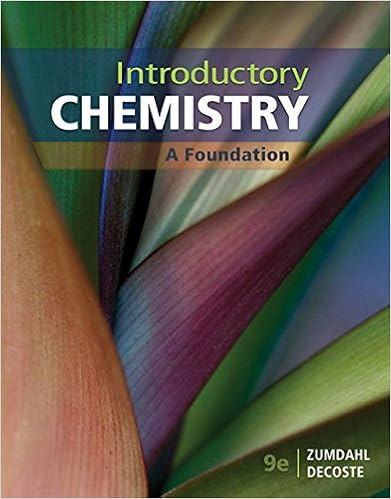# Section ii calculations please make sure you show all

• Test Prep
• 7

This preview shows page 5 - 7 out of 7 pages.

##### We have textbook solutions for you!
The document you are viewing contains questions related to this textbook.The document you are viewing contains questions related to this textbook.
Chapter 7 / Exercise 20
Introductory Chemistry: A Foundation
ZumdahlExpert Verified
and whether the reaction is endothermic or exothermic. Section II: CalculationsPlease make sure you show ALL of your work!!!! ALL ANSWERS SHOULD INCLUDE UNITS AND SIGNIFICANT FIGURES.28. At constant temperature, a sample of helium gas expands from an initial volume of 922 mL to 1.14 L against a pressure of 2.33 atm. Find w (in Joules) done by thegas (101.3 J = 1 atm*L) (5)-51.5 J
##### We have textbook solutions for you!
The document you are viewing contains questions related to this textbook.The document you are viewing contains questions related to this textbook.
Chapter 7 / Exercise 20
Introductory Chemistry: A Foundation
ZumdahlExpert Verified
29. One piece of copper jewelery at 105°C has twice the mass of another piece at 45°C. Both are placed in a calorimeter of negligible heat capacity. What is the final temperature inside the calorimeter (CCu= 0.387 J/g°C) (5)85 °C30. Tums are taken to reduce acid in the stomach. Calcium carbonate (Tums) is reacted with 1.0 M hydrochloric acid in the stomach. Carbon dioxide, water and calcium chloride are formed. (SHOW ALL WORK)a.Write the balanced chemical equation for reaction. (1)b.Write the balanced chemical equations for the formation of each compound from its constituent elements. (5) (Show that they all sum to the balanced chemical equation in part a.)
c.Using the following table of ΔH°fto determine the ΔHrxn. Indicate if the reaction is exothermic or endothermic. (10)
d.If you take a 1000 mg tablet, how much energy would be evolved in the reaction with excess hydrochloric acid in your stomach. (4)31. A laser pulse with wavelength 555 nm contains 4.85 mJ of energy. How many photons are in the laser pulse? (6)
•••